Download Presentation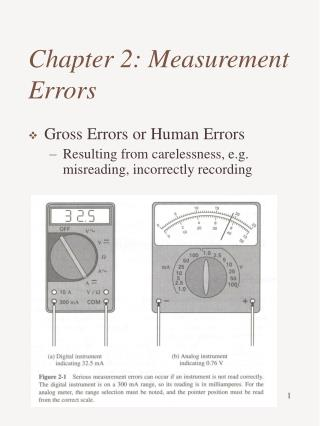Chapter 2: Measurement Errors

# Chapter 2: Measurement Errors - PowerPoint PPT Presentation

Chapter 2: Measurement Errors. Gross Errors or Human Errors Resulting from carelessness, e.g. misreading, incorrectly recording. Systematic Errors Instrumental Errors Friction Zero positioning Environment Errors Temperature Humidity Pressure Observational Error Random Errors.I am the owner, or an agent authorized to act on behalf of the owner, of the copyrighted work described.
Download Presentation## Chapter 2: Measurement Errors

An Image/Link below is provided (as is) to download presentation

Download Policy: Content on the Website is provided to you AS IS for your information and personal use and may not be sold / licensed / shared on other websites without getting consent from its author.While downloading, if for some reason you are not able to download a presentation, the publisher may have deleted the file from their server.

- - - - - - - - - - - - - - - - - - - - - - - - - - E N D - - - - - - - - - - - - - - - - - - - - - - - - - -
Presentation Transcript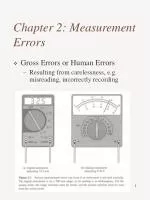Chapter 2: Measurement Errors
• Gross Errors or Human Errors
• Resulting from carelessness, e.g. misreading, incorrectly recording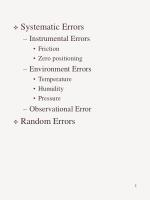Systematic Errors
• Instrumental Errors
• Friction
• Zero positioning
• Environment Errors
• Temperature
• Humidity
• Pressure
• Observational Error
• Random Errors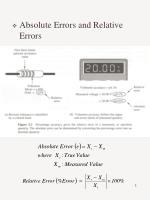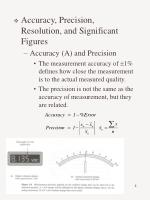Accuracy, Precision, Resolution, and Significant Figures
• Accuracy (A) and Precision
• The measurement accuracy of 1% defines how close the measurement is to the actual measured quality.
• The precision is not the same as the accuracy of measurement, but they are related.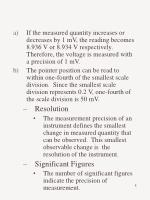If the measured quantity increases or decreases by 1 mV, the reading becomes 8.936 V or 8.934 V respectively. Therefore, the voltage is measured with a precision of 1 mV.
• The pointer position can be read to within one-fourth of the smallest scale division. Since the smallest scale division represents 0.2 V, one-fourth of the scale division is 50 mV.
• Resolution
• The measurement precision of an instrument defines the smallest change in measured quantity that can be observed. This smallest observable change is the resolution of the instrument.
• Significant Figures
• The number of significant figures indicate the precision of measurement.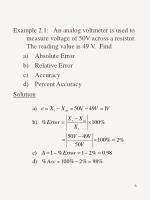Example 2.1: An analog voltmeter is used to measure voltage of 50V across a resistor. The reading value is 49 V. Find
• Absolute Error
• Relative Error
• Accuracy
• Percent Accuracy

Solution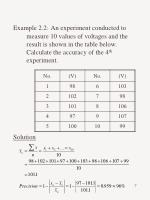Example 2.2:An experiment conducted to measure 10 values of voltages and the result is shown in the table below. Calculate the accuracy of the 4th experiment.

Solution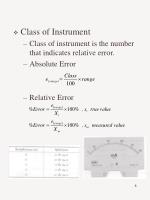Class of Instrument
• Class of instrument is the number that indicates relative error.
• Absolute Error
• Relative Error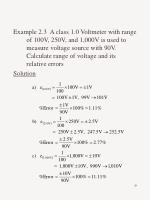Example 2.3 A class 1.0 Voltmeter with range of 100V, 250V, and 1,000V is used to measure voltage source with 90V. Calculate range of voltage and its relative errors

Solution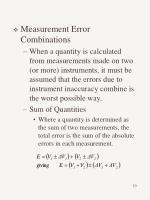Measurement Error Combinations
• When a quantity is calculated from measurements made on two (or more) instruments, it must be assumed that the errors due to instrument inaccuracy combine is the worst possible way.
• Sum of Quantities
• Where a quantity is determined as the sum of two measurements, the total error is the sum of the absolute errors in each measurement.Difference of Quantities
• The error of the difference of two measurements are again additive
• Product of Quantities
• When a calculated quantity is the product of two or more quantities, the percentage error is the sum of the percentage errors in each quantityQuotient of Quantities

• Quantity Raised to a Power
• Example 2.4 An 820Ω resistance with an accuracy of carries a current of 10 mA. The current was measured by an analog ammeter on a 25mA range with an accuracy of of full scale. Calculate the power dissipated in the resistor, and determine the accuracy of the result.Solution
• Basics in Statistical Analysis
• Arithmetic Mean Value
• Minimizing the effects of random errorsDeviation
• Difference between any one measured value and the arithmetic mean of a series of measurements
• May be positive or negative, and the algebraic sum of the deviations is always zero
• The average deviation (D) may be calculated as the average of the absolute values of the deviations.Standard Deviation and Probable of Error
• Variance: the mean-squared value of the deviations
• Standard deviation or root mean squared (rms)
• For the case of a large number of measurements in which only random errors are present, it can be shown that the probable error in any one measurement is 0.6745 times the standard deviation:Example 2.5 The accuracy of five digital voltmeters are checked by using each of them to measure a standard 1.0000V from a calibration instrument. The voltmeter readings are as follows: V1 = 1.001 V, V2 = 1.002, V3 = 0.999, V4 = 0.998, and V5 = 1.000. Calculate the average measured voltage and the average deviation.

Solution